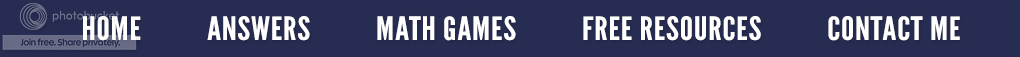### Patterns and Problem Solving

In my last two posts, I have presented some patterns for you to look at with the hopes that you would try to dissect them and be able to answer a few questions.  Are you ready for two more?  Here is the first one that I call Consecutive Number Series.

What counting pattern do you see in this sequence?  How would you describe the sequence of numbers that are being added?  What pattern do you see in the answers?  Can you figure out the pattern for 8 × 8 and 9 × 9?  Notice this pattern made a triangle.  Do you know what kind it is?

My next pattern I call The Eights, and you will readily see why. It, too, forms a triangle, but a different kind. Do you recognize the triangle as isosceles?  If you take a ruler, you will find that the base is 4" while the sides are both 3".

What do you think will happen if we take this same pattern and add a 0?  Notice that this pattern does not begin with adding an eight.  Can you figure out why?

I use this type of patterns with my remedial math college students because I consider it important to do some problem solving while recognizing and describing patterns.  After all, problem solving is a part of life.  It doesn't occur in a vacuum. Because students must reason about some specific content, I think patterns are a great place to begin.  Problem solving also helps students to make connections to other parts of mathematics and find some relevance to what they are learning.  And did you know, that problem solvers are typically better test takers?  So take the patterns from my last three postings, and create some of your own questions for your students.  Use them in a journal or as a small group activity.  But whatever you do, have fun learning and discovering patterns in math.

### The Beauty of Math Patterns

Some people say mathematics is the science of patterns which I think is a pretty accurate description. Not only do patterns take on many forms, but they occur in every part of mathematics. But then again patterns occur in other disciplines as well. They can be sequential, spatial, temporal, and even linguistic.

Recognizing number patterns is an important problem-solving skill. If you recognize a pattern when looking systematically at specific examples, that pattern can then be used to make things easier when needing a solution to a problem.

Mathematics is especially useful when it helps you to predict or make educated guesses, thus we are able to make many common assumptions based on reoccurring patterns. Let’s look at our first pattern below to see what we can discover.

What can you say about the multiplicand? (the number that is or is to be multiplied by another. In the problem 8 × 32, the multiplicand is 32.)  Did you notice it is multiples of 9?  What number is missing in the multiplier?
Now look at the product or answer.  That’s an easy pattern to see!  Use a calculator to find out what would happen if you multiplied 12,345,679 by 90, by 99 or by 108? Does another pattern develop or does the pattern end?
Here is a similar pattern that uses the multiples of 9.  How is the multiplier in this pattern different from the ones in the problems above?  Look at the first digit of each answer (it is highlighted).  Notice how it increases by 1 each time.  Now, observe the last digit of each answer. What pattern do you see there?  Using a calculator, determine if the pattern continues or ends.
Recognizing, deciphering and understanding patterns are essential for several reasons. First, it aids in the development of problem solving skills. Secondly, patterns provide a clear understanding of mathematical relationships. Next, the knowledge of patterns is very helpful when transferred into other fields of study such as science or predicting the weather.  But more importantly, understanding patterns provides the basis for comprehending Algebra since a major component of solving algebraic problems is data analysis which, in turn, is related to the understanding of patterns. Without being able to recognize the development of patterns, the ability to be proficient in Algebra will be limited.
So everywhere you go today, look for patterns.  Then think about how that pattern is related to mathematics. Better yet, share the pattern you see by making a comment on this blog posting.